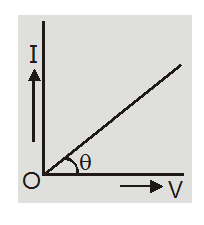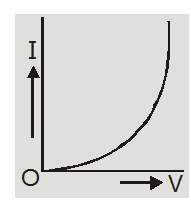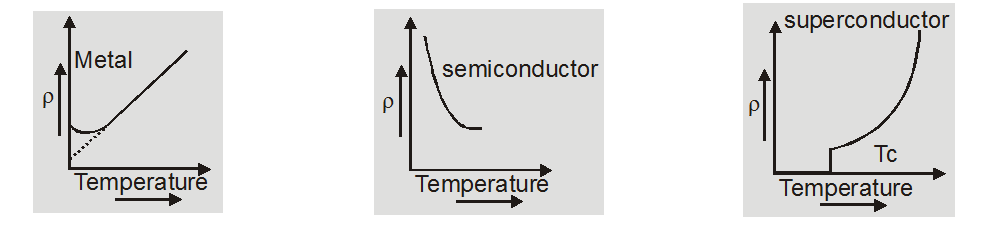# State and explain ohm's law - Ohm’s law and resistance - eSaral

Hey, do you want to learn about How to state and explain ohm's law? If yes. Then keep reading.

## Ohm’s Law:

If the physical state i.e. temperature, nature of the material, and dimensions of a conductor remain unchanged then the ratio of the potential difference applied across its ends to the current flowing through it remains constant.

$V \propto I$

Or

$\mathrm{V}=\mathrm{I} \mathrm{R}$

where $R=\frac{V}{I}$ is resistance of conductor.

$I=n$ e $A v_{d}$

$=n$ e $A \frac{e E}{m} \tau=\left(\frac{n e^{2} \tau}{m}\right)$

$\mathrm{AE}=\left(\frac{\mathrm{ne}^{2} \tau}{\mathrm{m}}\right) \mathrm{A} \frac{\mathrm{V}}{\mathrm{L}}$

So

$\mathrm{R}=\frac{\mathrm{V}}{\mathrm{I}}=\left(\frac{\mathrm{m}}{\mathrm{ne}^{2} \tau}\right) \frac{\mathrm{L}}{\mathrm{A}}$

R is resistance of conductor

### Important Points

1. The property of a substance due to which it opposes the flow of current through it is called resistance.

2. It is a scalar quantity with a unit volt/ampere called ohm $(\Omega)$dimensions of $\mathrm{R}=\frac{\mathrm{V}}{\mathrm{I}}$$=\frac{\mathrm{W}}{\mathrm{qI}}=\frac{\mathrm{M}^{1} \mathrm{~L}^{2} \mathrm{~T}^{-2}}{\mathrm{ATA}} =\mathrm{M}^{1} \mathrm{~L}^{2} \mathrm{~T}^{-3} \mathrm{~A}^{-2} 3. The reciprocal of resistance is called conductance G=\frac{1}{R}The SI unit is \mathrm{ohm}^{-1} or siemen (s) and its dimensions are \mathrm{M}^{-1} \mathrm{~L}^{-2} \mathrm{~T}^{3} \mathrm{~A}^{2} 4. The substances which obey ohm’s law are called ohmic or linear conductor. The resistance of such conductors is independent of the magnitude and polarity of applied potential differences. Here the graph between I and V is a straight line passing throughthe origin. The reciprocal of slope of straight line gives resistance R=\frac{V}{I}=\frac{1}{\tan \theta}= constante.g. silver, copper, mercury, carbon, mica, etc. 5. The substances which do not obey ohm’s law are called nonohmic or nonlinear conductors. The I–V curve is not a straight line.i.e. p n diode, transistors, thermionic valves, rectifiers, etc.6. V = IR defines resistance and hence it is applicable to all ohmic and nonohmic conductors. If R is constant or V \propto I then it represents ohm’s law. 7. The relation R=\frac{V}{I} is macroscopic form while \rho=\frac{E}{J} is microscopic form of ohms law. 8. The resistance of a conductor depends on temperature, nature of material, length and area of cross-section. 9. The temperature dependence of resistance is given by R=R_{0}(1+\alpha \Delta \theta) where \alpha is temperature coefficient of resistance and \Delta \theta is change in temperature. The variation is graphically represented as.The temperature coefficient of resistance \alpha=\frac{R-R_{0}}{R_{0} \Delta \theta} is defined as change in resistance per unit resistance at 0^{\circ} \mathrm{C} per degree rise of temperature. 10. For maximum metals \alpha=\frac{1}{273} per { }^{\circ} \mathrm{C} so \mathrm{R}=\mathrm{R}_{0}\left(1+\frac{\Delta \theta}{273}\right)$$=\mathrm{R}_{0}\left(\frac{273+\Delta \theta}{273}\right)$

$=\mathrm{R}_{0} \frac{\mathrm{T}}{273}$

so $R \propto T$

i.e. The resistance of pure metallic conductor is proportional to its absolute temperature.
11. $R \propto L$ and $\mathrm{R} \propto \frac{1}{\mathrm{~A}}$so$R \propto \frac{L}{A}$ or
$R=\rho \frac{L}{A}$

where $\rho$ is called resistivity or specific resistance.

12. The fractional change in resistance without a change in volume or mass are:

(a) When change in length is small $(\leq 5 \%)$ fractional change in $\mathrm{R}$ is$\frac{\Delta R}{R}=\frac{2 \Delta L}{L}$

(b) When change in radius is small $(\leq 5 \%)$ fractional change in R is

$\frac{\Delta R}{R}=\frac{-4 \Delta r}{r}$

(c) When change in area is small $(\leq 5 \%)$ fractional change in R is

$\frac{\Delta \mathrm{R}}{\mathrm{R}}=\frac{-2 \Delta \mathrm{A}}{\mathrm{A}}$

1. In terms of microscopic quantities, $E=\rho J$ so resistivity is numerically equal to the ratio of the magnitude of the electric field to current density.

2. $\rho=\frac{R A}{L}$ so if L = 1 m, $A=1 \mathrm{~m}^{2}$ then $\rho=R$. Specific resistivity is numerically equal to the resistance of substance having a unit area of cross-section and unit length.

3. It is a scalar with unit ohm-meter $(\Omega-\mathrm{m})$ and dimensions $M^{1} L^{3} T^{-3} A^{-2}$

4. The reciprocal of resistivity is called conductivity or specific conductance with units mho/m dimensions$\mathrm{M}^{-1} \mathrm{~L}^{-3} \mathrm{~T}^{3} \mathrm{~A}^{2}$ $\sigma=\frac{1}{\rho}=\frac{n e^{2} \tau}{m}=$ ne $\mu$

5. The resistivity is independent of the shape and size of the body and depends on the nature of the material of the body. The resistivity is the property of material while resistance is the property of the object.

6. The temperature dependence of resistivity is given by relation $\rho=\rho_{0}(1+\propto \Delta \theta)$ where $\propto$is the temperature coefficient of resistivity and $\Delta \theta$ is change in temperature. For metals, $\alpha$ is positive so resistivity increases with temperature while for non-metals $\alpha$ is negative so resistivity decreases with temperature.### Specific use of conducting materials:

1. The heating element of devices like heater, geyser, press, etc are made of nichrome because it has high resistivity and high melting point. It does not react with air and acquires a steady state when red hot at 800 ${ }^{\circ} \mathrm{C}$.

2. Fuse wire is made of tin-lead alloy because it has a low melting point and low resistivity. The fuse is used in series and melts to produce an open circuit when the current exceeds the safety limit.

3. Resistances of resistance boxes are made of manganin or constantan because they have moderate resistivity and a very small temperature coefficient of resistance. The resistivity is nearly independent of temperature.

4. The filament of the bulb is made up of tungsten because it has low resistivity, a high melting point of 3300 K, and gives light at 2400 K. The bulb is filled with inert gas because at high temperature it reacts with air forming oxide.

5. The connection wires are made of copper because it has low resistance and resistivity.

so, that's all from this article. I hope you get the idea about How to state and explain ohm's law. If you found this article informative then please share it with your friends. If you have any confusion related to this topic then feel free to ask in the comments section down below.

For a better understanding of this chapter, please check the detailed notes of Current Electricity. To watch Free Learning Videos on physics by Saransh Gupta sir Install the eSaral App.